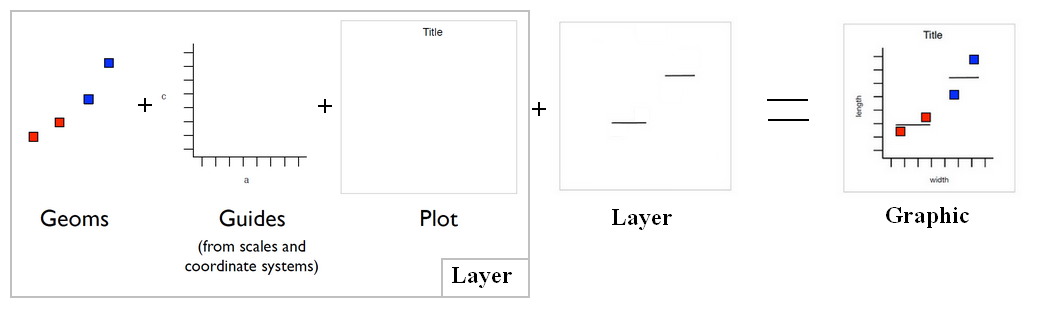# Ggplot - Smooth (Geom_Smooth|Stat_Smooth)

smoothing in Ggplot

Smooth (Smoothed conditional means) is seen as a:

• stat - mean calculation
• and a geom - line or point

stat_smooth() is a layer and an alias of geom_smooth(). They will add a line of best fit to a plot.

## Function / Method

Smoothing method (function) to use, eg. “lm”, “glm”, “gam”, “loess”, “rlm”.

Auto = loess is used for less than 1,000 observations; otherwise gam is used with formula = y ~ s(x, bs = “cs”). loess gives a better appearance, but is O(n^2) in memory, so does not work for larger datasets.

Recommended PagesGGplot - Layer (data + mapping + geom + stat + position)

Layer = data: The data frame (in a variable or a function, if null inherited) + mapping: Mapping data to geom properties (ie aesthetics) + geom : The geometric object to use display the data +...